Faydi, Y., Brancheriau, L., Pot, G., and Collet, R. (2017). "Prediction of oak wood mechanical properties based on the statistical exploitation of vibrational response," BioRes. 12(3), 5913-5927.

Abstract

In the European Union, timber is used in structural applications and must be graded with a Conformité Européene (CE) mark. To achieve standard, machine strength grading is used. A common technology for these machines is based on using the vibrational response of each wood board to estimate the timber modulus of elasticity and modulus of rupture. The first Eigen frequency is usually used to predict these mechanical properties. However, in heterogeneous wood species such as oak, this parameter is less correlated with mechanical properties. The current study proposes two new methods based on an extended exploitation of the vibrational response that predicts oak wood mechanical properties. The first method was based on the mechanical parameters deduced from several Eigen frequencies that were chosen with regards to a stepwise regression. The second method was based on the full vibrational spectrum and used a partial least squares method. The first method slightly improved the prediction of the modulus of elasticity compared with the first Eigen frequency in edgewise transversal vibration. Both methods significantly improved the prediction of the modulus of rupture.

Prediction of Oak Wood Mechanical Properties Based on the Statistical Exploitation of Vibrational Response

Younes Faydi,a,* Loic Brancheriau,b Guillaume Pot,a and Robert Collet a

In the European Union, timber is used in structural applications and must be graded with a Conformité Européene (CE) mark. To achieve standard, machine strength grading is used. A common technology for these machines is based on using the vibrational response of each wood board to estimate the timber modulus of elasticity and modulus of rupture. The first Eigen frequency is usually used to predict these mechanical properties. However, in heterogeneous wood species such as oak, this parameter is less correlated with mechanical properties. The current study proposes two new methods based on an extended exploitation of the vibrational response that predicts oak wood mechanical properties. The first method was based on the mechanical parameters deduced from several Eigen frequencies that were chosen with regards to a stepwise regression. The second method was based on the full vibrational spectrum and used a partial least squares method. The first method slightly improved the prediction of the modulus of elasticity compared with the first Eigen frequency in edgewise transversal vibration. Both methods significantly improved the prediction of the modulus of rupture.

Keywords: Wood mechanical properties; Vibration test; Partial least squares; Stepwise regression; Oak

Contact information: a: LaBoMaP, Arts et Metiers, HESAM, COMUE BFC, 71250 Cluny FRANCE; b: CIRAD – Département PERSYST, UPR 114 “Biomasse, bois, énergie, bio-produits” TA B114/16 73 Rue Jean François Breton 34398 Montpellier Cedex 5; *Corresponding author: younes.faydi@ensam.eu

INTRODUCTION

The characterization of timber mechanical properties is needed for structural applications of wood. However, wood heterogeneity requires the individual characterization of each board. European standards have defined different grades based on three characteristics (EN 338 (2016); EN 384 (2016)): density, modulus of elasticity (MOE), and modulus of rupture (MOR). The reference for these properties is based on a four point bending test according to the EN 408 (2012) standard. However, in the frame of machine strength grading described in EN 14081 (2016), these properties must be determined nondestructively. Different mechanical grading methods and machines are commercially available and based on technologies including X-rays, vibrational methods, or stress ratings (Hanhijarvi et al. 2005; Baillères et al. 2012). Mechanical properties are typically predicted from the board’s density or dynamic measurement of the MOE, and sometimes with the help of the detection of singularities like knots or fiber direction.

The board mass and dimensions allow for the measurement of the global board density. MOE can also be estimated by dynamic methods (Hanhijarvi et al. 2005; Baillères et al. 2012). The most challenging characteristic to predict is the MOR. The efficiency of prediction is typically evaluated with the coefficient of determination (R²) between indicating properties and destructively obtained variables. However, it is also essential to estimate the mean error of prediction to evaluate each model. The coefficient of determination between the measured MOR and MOR indicating property obtained by non-destructive techniques can reach R² = 0.68 for Spruce and R² = 0.58 for Douglas fir (Viguier et al. 2015). However, there is little data available in the literature concerning the prediction of hardwood mechanical properties and particularly oak wood. It could be mentioned, nonetheless, that the coefficient of determination between static MOE and MOR for chestnut was R² = 0.36 in Vega et al. (2013), which is lower than spruce with R² = 0.71 in Viguier et al. (2017). Grading oak is a major difficulty for the large commercialization of structural applications (Collet et al. 2011). Thus, more effort is needed to improve its characterization and non-destructive grading.

This paper describes the use of vibrational analysis to predict MOE and MOR. The first Eigen frequency allows the prediction of the MOE (Hanhijarvi et al. 2005; Baillères et al. 2012). Guindos and Guaita (2013, 2014) showed that MOR depends on knot characteristics including shape, position, etc. Detecting these characteristics is essential for MOR prediction. Roohania et al. (2015) observed the influence of the position and orientation of a local heterogeneity on the resonance frequencies by comparing clear beams and beams that were drilled. The behavior of the free flexural vibration was different depending on the position and orientation of the heterogeneity. When the drilling axis lies in the vibration bending plane, the weakening of frequency was maximal at the location of an antinode of vibration. However, the frequency offset was maximal in the vibration node when the drilling axis was orthogonal to the bending plane. This particular behavior could be used for grading lumber because it takes into account the presence of defects by the estimation of the static MOE and the MOR. For the same purpose, Sobue et al. (2010) detected the position of defects by looking at the resonance frequency shifts in longitudinal vibrating. Indeed, the shift was large when the defect coincided with a node of vibration, while no shift was observed when the defect coincided with an antinode of vibration. The proposed method worked only to identify a maximum of two dominant defects. Another study from Yang et al. (2002) detected some defects such as knots by comparing clear and defected boards. The criterion used was the variation of the mode shape of transversal vibration waves. The theoretical and experimental mode shapes coincided with clear beams and differed near a defect. None of the previous studies went beyond heterogeneity detection to predict MOR from vibrational signals.

The current study proposed two analysis techniques based only on vibrational measurements to predict MOE and MOR. The first method was based on a stepwise regression and considered several output parameters from the vibration test. Stepwise regression detects the most representative parameters that explain most of the variance in MOE and MOR. The second technique was related to frequency spectrum standardization in frequency and in amplitude. In the second method, the partial least squares (PLS) statistical method was used, such that each frequency of the spectrum was a predictive variable. Brancheriau and Baillères (2003) conducted a similar analysis for larch species, but they did not standardize the frequency vectors. Thus, their analysis was dependent on the board dimensions. The current study standardized the amplitude and the frequency, which resulted in the removal of the percussion impact effect and board dimensions effect. These two analysis techniques were compared to the more classical use of the dynamic MOE, based on the first Eigen frequency in longitudinal vibration as an indicating property of static MOE and MOR.

EXPERIMENTAL

Sampling

A total of 164 boards of French oak wood (Quercus petraea and Quercus robur) were tested in normal laboratory conditions of 20 °C and 65% humidity as noted in the EN 408 (2012) standard. The boards were selected according to the NF B52 001-1 (2011) visual grading standard to ensure an almost equal distribution between the three grades of D30, D24, and D18 (Table 1). The waste category was over-represented because the goal of machine grading is to reduce the underestimation of visual grading. Four different board dimensions were chosen (Table 2). The number of boards was different in each section because the wood board manufacturing process reduced the final number of exploitable boards.

Apparatus

Four points bending

A four point bending test was performed according to EN 408 (2012) using the distance between edge supports equals to 18 times the board’s height, and a distance between the central loading heads equals to 6 times the board’s height. These distances were modified according to each cross-section. The longitudinal position of the boards was chosen so that the supposed critical board’s zone was between loading heads. The tension edge was selected at random. Global MOE and MOR were determined in accordance with the EN 408 (2012) standard and constituted the observations for statistical methods.

Table 1. Board Grading based on Visual Method (NF B52 001-1 (2011))Table 2. Board Numbers for Each SectionVibrational measurements

Vibration tests were carried out using the BING device developed by CIRAD (Brancheriau et al. 2007). The technique consisted of generating an impulse with a hammer in beam extremity and placing a microphone as a receiver on the other side. The beam was placed on two elastic supports to ensure free vibrations. These supports have been positioned at the theoretical node points at the first mode in bending. Three types of vibrational tests were done. An impact along the sample involved longitudinal vibrations representative of a compressive test (Fig. 1); bending or transversal vibrations were generated by edgewise and flatwise impacts (Fig. 1). The longitudinal vibration test represents industrial measurements of the dynamic MOE using the first Eigen frequency. The analog output signal was converted to a digital one with a sampling frequency of 39 kHz, acquisition of 1678 ms, and 12 bits resolution. A discrete Fourier transformation converted the temporal response to a frequency spectrum.

Method Based on Eigen Frequencies

Global output parameters

The relationship between Eigen frequencies and MOE or shear modulus depend on the vibration direction and the mechanical model applied. Several output parameters were computed from these different relationships and used later as predictive variables. Equations 1, 2 and 3 were demonstrated in Brancheriau and Bailleres (2002), who made a review and theoretical comparison of the different models.

In longitudinal vibrations, the MOE is computed thanks to the first Eigen frequency only, following Eq. 1,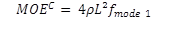(1)

where MOEcis the MOE in longitudinal vibrations (compression), is the density, L is the length, and is the frequency of the first mode in longitudinal vibration.

In transversal vibrations (edgewise and flatwise), four Eigen frequencies were chosen. In this case, two common theories were used to determine the MOE: Bernoulli (1748) and Timoshenko (1921). Bernoulli theory does not consider beam shearing. To compute the MOE according to Bernoulli theory, equation 2 was used,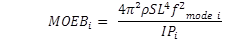(2)

Where MOEBis the MOE following Bernoulli theory for the ith mode in transversal vibrations; p is the density; L is the length;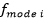is the frequency of the ith mode in transversal vibration; S is the cross section; is the second moment of area; Pis a scalar relative to the ith mode (ith solution of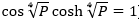). Applying Timoshenko bending theory, Bordonné (1989) and more recently Brancheriau and Bailleres (2002) gave the following solution of the equation of motion for a beam in transversal vibration,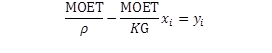(3)

where MOET is the MOE following Timoshenko theory, p is the density, K is the shear factor (K=5/6 for a rectangular cross-section), G is the shear modulus, xi and yi are parameters that depend on the Eigen frequency (Brancheriau and Bailleres (2002) for detailed expression). Thanks to a linear regression between xi and yi parameters for the four selected Eigen frequencies, the MOE and shear modulus were found. The coefficient of determination of these regressions were also computed to be used as predictive variables (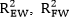in Table 3).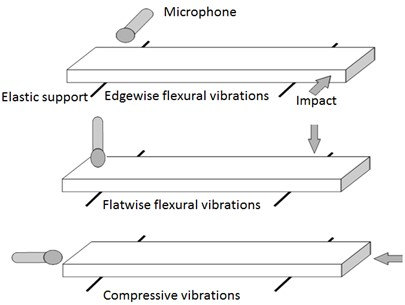Fig. 1. Illustration of vibrational measurements

As suggested by Roohania et al. (2015), a ratio between edgewise and flatwise vibrational MOE was computed for each mode. The inharmonicity factor, proposed by Aramaki et al.(2007), was computed. It represented the ratios between the second, third, and fourth Eigen frequencies divided by the first Eigen frequency. To take into account the viscoelastic behavior, the loss factor (tan δ) was determined for the first Eigen frequency (Brancheriau et al. 2010). The loss factor could have been calculated for each Eigen frequency, but the first was the only one that was considered because of its high energy and low computational uncertainty. Finally, it was possible to extract 27 variables, as presented in Table 3. This list of parameters organized the input data for the stepwise regression method.

Table 3. List of Global Output Vibrational Variables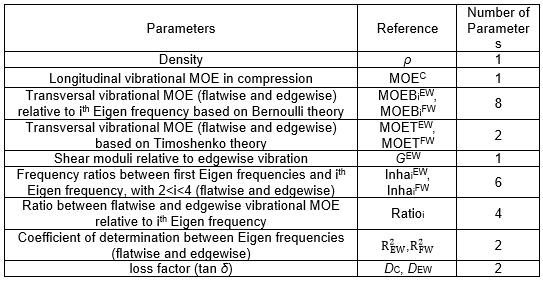Stepwise regression

The stepwise regression (Ing and Lai 2011) aimed to fit a model based on the vibrational parameters of Table 3 to predict the static MOE and MOR. The stepwise regression was conducted with R software (Venables and Smith 2017) with the Akaike information criterion method described by Venables and Ripley (2002).

Method Based on the Full Vibrational Spectrum

This analysis considers standardizing amplitudes of the whole spectrum for each vibration test as predictive variables. Thus, the spectrum was standardized in its frequencies and amplitudes. Note that the present method only used the edgewise vibrational measurements for reasons that are discussed later.

Standardization of spectrum frequencies

The determination of the fundamental frequency f0 allowed standardized frequencies by computing the ratio of each frequency f to the fundamental frequency. The standardization made the analysis independent from the board’s dimensions. Thus, each board had its own frequency scale (f/f0). By using a linear interpolation, only one frequency scale was constituted for all measured spectra.

Standardization of spectrum amplitudes

Explicative variables were composed of amplitudes which were standardized to remove impact influence. The signal energy was defined as follows (Eq. 4),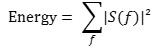(4)

where S(f) is the magnitude of the Fourier transform at the frequency f. In a linear system, the responses of the system under two different impacts were as follows,(5)

where S is the Fourier transform of the output signal, E is the Fourier transform of the input signal (impact), and H is the impulse response of the linear system. Assuming there is a linear relationship between E1 and E2 (α a constant):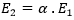, the output spectrum is then equal to: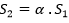and the signal energy: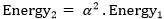. To remove the influence of impact, an appropriate standardization is to divide each spectrum amplitude by the square root of the energy: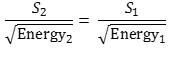(6)

Because this expression is non-dimensional, the MOE calculated from the first Eigen frequency extracted from edgewise tests was included in the final amplitude standardization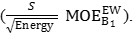The explicative and explained (MOE and MOR) variables were, in turn, in the same unit.

Partial least squares

The partial least squares (PLS) were applied on treated spectral signals to predict MOE and MOR. The PLS was based on multiple linear regressions (MLR) represented by Eq. 7. MLR looks at a linear relationship between explicative variables (elements of the spectrum) to explain a single dependent goal variable (MOE or MOR) according to Eq. 7, which is an example for MOR,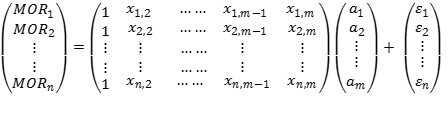(7)

where MORi is the ith observation of goal variable, n is the number of observations, m is the number of explicative variables, xi,j is the jth standardized amplitude of spectrum in the ithobservation, ais the jth element of regression coefficient and ɛi is the ith residual element.

The goal was to avoid the collinearities between the variables by compressing the information and maximizing the explained variance of the dependent variable (MOE or MOR). Equation 7 was then converted to Eq. 8. The details of this method are available in Naes et al. (2002). Equation 8 is an example of two latent variables that are selected in the case of MOR.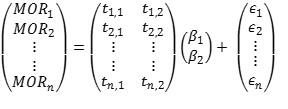(8)

Where MORi is the ith observation of goal variable, ti,j is the jth element of the ith latent variable which is a linear combination of initial explicative variables, βis the jth new coefficient element of regression and ɛi is the ith residual element.

The number of latent variables was selected in order to minimize the error between the predicted and actual values of the goal variable (cross validation with 10 random segments). The population was separated into ten random folds. Calibrations and validations were done ten times with different samples. Calibration was completed with 9 folds, while validation was completed with the last one. The principle is detailed in the publication of Næs et al. (2002). After cross validation, the root mean square error of cross validation (RMSECV) was calculated (Eq. 9). The selected number of latent variables correspond to the lowest RMSECV. The associated coefficient of determination of cross validation (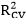) was also determined.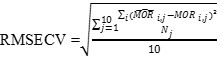(9)

where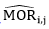is the ith estimate value of MORi.j at the jth fold of cross validation (note that the same equation was used for MOE), and Nj is the number of samples used in validation (jthfold).

The final model was computed using all the observations. The root mean square error of calibration (RMSEC) was defined on the total number of observations (n) as follows,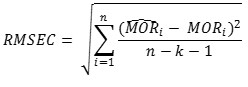(10)

where k is the number of latent variables and n is the total number of observations. The computation was performed with R software with the PLS package (Mevik and Wehrens 2007).

RESULTS AND DISCUSSION

Comparison of Destructives and Nondestructive Tests

Table 4 shows the descriptive statistics of density, MOE, and MOR. Destructive tests showed a large representation of high MOR values (MOR > 30 MPa), which constitutes 88% of the total number of boards. However, boards with a low MOR were not negligible. Indeed, 12% of boards were under 30 MPa.

Table 4. Statistics of Density, MOE, and MOR Obtained by Destructive Tests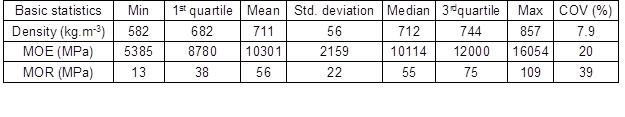Despite the large range between the maximum and minimum values of density (275 kg.m-3), its dispersion, which is represented by the coefficient of variation (COV), was low (COV = 7.9%) with a mean value of 711 kg.m-3. Close values were observed in the PhD of Viguier (2015) where the mean of density equals to 725 kg.m-3 and the COV = 9.3%.

The dispersion of MOE was more pronounced than the density with a COV = 20%, a range of 10669 MPa, and a mean value of 10301 MPa. The values observed in the PhD of Viguier (2015) where the mean of MOE equals to 10140 MPa and the COV = 24.8% are close to those obtained in this study.

The same observation was made for MOR with a large dispersion (COV = 39%), a range of 96 MPa and a mean value of 56 MPa. The mean value of MOR observed in the PhD of Viguier (2016) were equals to 40.7 MPa and the COV% = 37.8% which are lower comparing to this study.

Coefficients of determination values (with interval of confidence p < 0.05) and their degree of significance between density, MOE, and MOR are shown in Fig. 2. The correlation between static MOE and density was low (R² = 0.09) with a large interval of confidence. Thus, predicting MOE from the density appears to be inappropriate. A similar observation was made between the density and static MOR (R² = 0.01). However, the r-squared value between the static MOE and static MOR was equal to 0.40. As a result, it was interesting to determine the MOE to predict the MOR by using non-destructive techniques.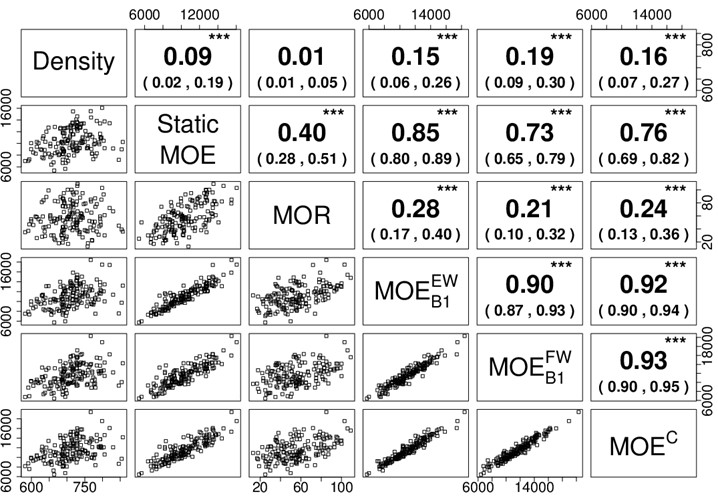Fig. 2. Scatterplots of density, static MOE, static MOR, and vibrational MOE. R-squared values are in the upper right panel, with the significance in upper case (*** for p-value <0.001, nothing for 0.1 < p-value < 1). The confidence intervals at the 0.05 level are shown in brackets.

Typically, grading machines based on vibration measurements use the dynamic MOE obtained from the first Eigen frequency in longitudinal vibration to determine MOE and MOR. In the current study, MOE and MOR were predicted from the dynamic MOE calculated by using transversal (edgewise vibrational MOE) and longitudinal (longitudinal vibrational MOE) vibration tests. A cross-validation was performed to estimate the prediction error of the statistical modeling. The models were stable in both cases of longitudinal and edgewise-transversal vibrations. Indeed, the RMSECV was close to the RMSEC. In the longitudinal vibration model, the prediction of MOE leads to a RMSEC of 1100 MPa and a RMSECV of 1035 MPa and the prediction of MOR leads to RMSEC of 19.1 MPa and a RMSECV of 19.2 MPa. The same behavior is observed in edgewise transversal vibrations model where the prediction of MOE leads to a RMSEC of 896 MPa and a RMSECV of 835 MPa and the prediction of MOR leads to RMSEC of 18.7 MPa and a RMSECV of 18.9 MPa. For both MOE and MOR prediction, the errors were higher for the longitudinal vibration than for the edgewise-transversal vibration.

Either in the longitudinal or transversal vibration, the vibrational method was a good way to predict the MOE for oak species (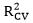> 0.75). In contrast, the MOR was not estimated accurately by the vibration tests (< 0.27). Thus, it is interesting to show how far spectrum analyses improve the prediction of MOE and MOR, first by using parameters based on Eigen frequencies, then the full spectrum.

Method based on Eigen Frequencies

This analysis used a stepwise regression method. The goal consisted of finding the best global output parameters that explain the variance of observations, i.e., MOE and MOR. Each model contained significant parameters with p-values that were under 0.001.

Stepwise regression for MOE

The selected regression parameters to predict MOE were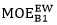and Ratio 2 which is the ratio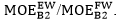. The determination coefficient of the regression was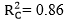(F-statistic = 496, p-value < 2.2 × 10-16) with a residual error of RMSEC = 812 MPa. The result of the cross-validation procedure was RMSECV = 817 MPa (= 0.86). This model was found to be stable because the RMSECV was very close to the RMSEC. Figure 3a shows the predicted values according to static MOE values.

The first selected parameter was the edgewise dynamic modulus, which corresponded to the elastic property measured in the same configuration than the static test (edgewise flexural loading). The second selected parameter was the second ratio between edgewise and flatwise dynamic moduli. This ratio was affected by the presence and positions of natural defects in the boards (Roohania et al. 2015). Due to the collinearity of the explicative variables, the first ratio () or the coefficient of determination between Eigen frequencies (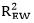) could be used too (in addition toparameter). By doing so, for the first ratio the model results were, RMSEC = 825 MPa, RMSECV = 830 MPa; and forthe model results were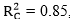, RMSEC = 831 MPa, RMSECV = 833 MPa.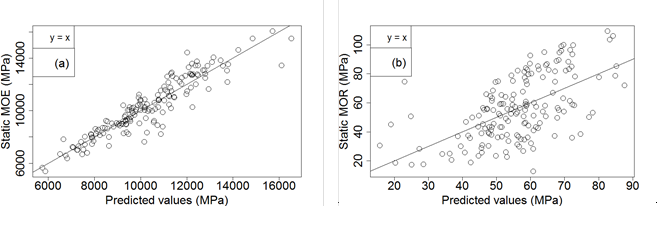Fig. 3. (a) Static MOE vs. predicted MOE. (b) Experimental MOR vs. predicted MOR. In both panels, the solid line is the first bisector.

Stepwise regression for MOR

The selected parameters to predict MOR were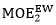and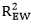. The regression coefficient was equal to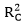= 0.35 (F-statistic = 42.8, p-value = 1.23 × 10-15), and the residual error was RMSEC = 18.0 MPa. The associated cross-validation gave a RMSECV value of 18.1 MPa (= 0.32). This model was stable because the RMSECV was close to the RMSEC. Figure 3b shows the regression scatterplot for the MOR values.

As well as the static MOE model, the first parameter selected was an edgewise elastic property (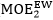). The second parameter was the coefficient of determination associated with the Bordonné’s solution of the Timoshenko equation (Brancheriau et al. 2002). This coefficient was affected by the values of the Eigen frequencies. If the material was perfectly homogeneous,would be equal to one. Otherwise, the presence and position of natural defects shifted the Eigen frequencies, lowering thevalue (Roohnia and Brancheriau 2015). The dynamic MOE corresponding to the first and second Eigen frequencies were correlated (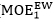andR-squared value of 0.95). Thus, replacingwithin the multiple regression withlead to similar results:= 0.34, RMSEC = 18.1 MPa, and RMSECV value of 18.2 MPa.

Method Based on the Full Vibrational Spectrum

Because multiple linear regressions showed that edgewise transversal vibration parameters were mainly selected to predict MOE and MOR, only the edgewise transversal vibrations were used in this section. The number of predictive variables (standardized frequencies) was reduced according to the bilateral R² threshold between the considered frequency and the dependent variable (MOE or MOR) in order to ensure stability to the model. We kept the frequencies associated with a bilateral R² greater than 0.025 (several R² thresholds were tested).

PLS regression for MOE

In regards to the bilateral R² criterion on the frequency selection, 597 frequencies were chosen for the MOE prediction (Fig. 4a). Figure 4a shows that the relevant frequencies kept for MOE prediction were below 10 f/f0. This corresponded to a maximum frequency range of 1250 Hz because the first Eigen frequency was 125 Hz maximum (average of 91 Hz). The bilateral R² value did not exceed 0.40. The selected frequencies were not only focused on the harmonic frequencies of the flexural vibrations but considers the whole spectrum. At low frequencies the vibration energy was at a maximum, but the wavelengths were large. Consequently it had little sensibility to punctual defects (high standardized frequencies were more sensible to punctual defects). However, the presence of natural defects might cause mode conversions from flexural to torsional and compressional vibrations, even in low frequencies.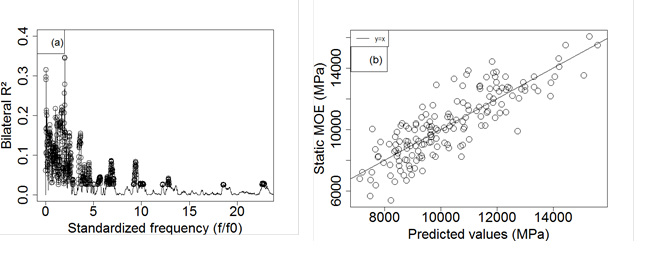Fig. 4. (a) Bilateral coefficient of determination between standardized frequencies and MOE. The hollow circles are the selected frequencies (R² ≥ 0.025). (b) Relationship between static and predicted MOE. Partial least squares regression on the full vibrational spectrum. The solid line is the first bisector.

Four latent variables were needed to minimize the root mean square error of cross validation (RMSECV = 1458 MPa,= 0.54). The partial least squares regression was significant with a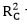value of 0.71 (F-statistic = 97.8, p-value < 2 × 10-16) and a RMSEC = 1175 MPa. However, the difference between the RMSECV and RMSEC highlighted the instability of the model (ratio of 1.24). The realistic error and determination coefficient of this model were those obtained by cross-validation. Figure 4b shows the reference MOE values according to predicted values of the partial least squares model.

PLS regression for MOR

The process of variable selection by bilateral R² was conducted to 290 selected frequencies (Fig. 5a). The selected frequencies were not all identical to those associated to the MOE. However, they remain 5 times lower than the first Eigen frequency, thus mostly below 625 Hz. For this model, thirteen observations were considered as outliers and were removed. The outlier detection was based on the distance of each observation to the centroid of the population computed in the basis defined by the selected scores (Euclidian distance applied in an orthogonal basis, which was equal to the Mahalonobis distance in this case).

To predict MOR, two latent variables minimized the error of cross-validation with a RMSECV value of 17.6 MPa (R²CV = 0.33). The partial least squares regression was significant with a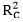value of 0.44 (F-statistic = 58.1, p-value < 2 × 10-16) and a RMSEC value of 16.25 MPa. RMSECV was found to be close to RMSEC with a ratio of 1.08. Figure 5b shows the reference MOR values according to the predicted values of the partial least squares model.

MOE and MOR predictive variables were obtained by multiplying the amplitudes of the frequency spectrum by the dynamic modulus. From a statistical point of view, there was only an interaction between the variables of amplitude and the dynamic modulus. The dynamic modulus was linked to the Eigen frequency, the density, and the dimensions. The amplitude of the vibrations depended on the internal viscosity of the material, the presence of natural defects, the wood stiffness, the positions of the supports, and the sensor. The interaction between these variables provided complementary information on the mechanical behavior of the wooden beams.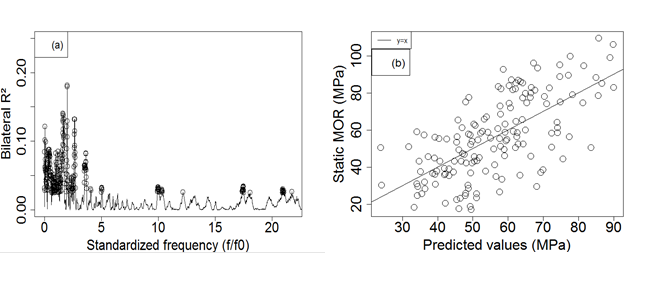Fig. 5. (a) Bilateral coefficient of determination between standardized frequencies and MOR. The hollow circles are the selected frequencies (R² ≥ 0.025). (b) Relationship between static and predicted MOR. Partial least squares regression on the full vibrational spectrum. The solid line is the first bisector.

Table 6. Summary of Prediction Methods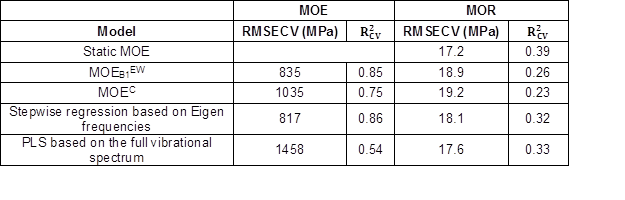Comparison of Models

Table 6 shows the results of MOE and MOR predictions for the different models in cross-validation. Concerning the MOE predictions, the stepwise regression method based on Eigen frequencies presented the lowest RMSECV, which equals 817 MPa. The associatedequals to 0.86, which demonstrates that this method explains a large part of the variance of MOE. However, a model based only on the first Eigen frequency in edgewise transversal vibration (MOEB1EW) presented similar values. This result was expected because MOEB1EW was the main variable used in the stepwise regression model. The model based on the first Eigen frequency in the longitudinal vibration (MOEC) presents a higher RMSECV value of 1035 MPa. The reason why the models based on MOEB1EW were the most efficient must be because the static MOE was measured in a bending test like MOEB1EW. It is interesting to notice that the longitudinal vibration is the more common method used in industry for practical reasons. Finally, the PLS method based on the full vibrational spectrum did not improve the prediction of MOE regarding RMSECV and(1458 MPa, 0.54, respectively).

For the MOR prediction, the PLS method based on the full vibrational spectrum conducted to the lowest RMSECV value of 17.6 MPa, which was similar to the one obtained when the static MOE was used as a predictive criterion. The correspondingvalue of 0.33 was greater than those of the others models. Indeed, with the stepwise regression method based on Eigen frequencies,equals 0.32 (RMSECV of 18.1 MPa). Using the first Eigen frequency in transversal vibration (MOEB1EW),equals 0.26 (RMSECV of 18.9 MPa). The first Eigen frequency in longitudinal vibration (MOEC) leads toof 0.23 (RMSECV of 19.2 MPa). As a result, the PLS method based on the full vibrational spectrum was better suited for MOR prediction. Indeed, the full spectrum contains the local information about wood defect (magnitudes taken by the frequency spectrum) which allows a better determination of MOR. But, the MOE is mainly dependent on the Eigen frequencies values, and this information was lost when the frequency scale was standardized this is why the stepwise regression method based on Eigen frequencies or the first edgewise transversal vibration (MOEB1EW) were better for the MOE prediction.

Because Since the PLS method was based on the full vibrational spectrum in edgewise transversal vibration, it is possible to build a single grading machine that can predict MOR and MOE with the bestvalues in a single measure. The obtained errors would be significantly lower than the current grading machine based on longitudinal vibration. For MOE prediction thewould increase from 0.75 to 0.86; and for MOR prediction thewould increase from 0.22 to 0.33. However, the PLS model is quite unstable due to the model sensibility to noise in the signals. To overcome this problem, specific filtering procedures and/or a signals average with multiple acquisitions can be tested. A larger experimental sample can also increase the robustness of the model.

CONCLUSIONS

1. By using transversal vibrations and statistical methods, it is possible to significantly improve MOE and MOR prediction with respect to the usual model based on longitudinal vibrations.
2. The stepwise regression method based on Eigen frequencies allowed an improvement of the MOE prediction by choosing specific global vibrational parameters. The main parameter is the dynamic MOE calculated from the first resonance frequency in the edgewise bending configuration. Concerning the MOR estimation, this method had a better efficiency than the usual models based only on the dynamic MOEs (bending or compression).
3. The PLS method based on the full vibrational spectrum did not improve the MOE prediction. However, the prediction of the MOR was very efficient and led to the lowest prediction error.
4. A combination of the two proposed statistical methods is possible in order to obtain the best prediction of MOE and MOR from a single edgewise vibrational measurement.

ACKNOWLEDGMENTS

This study was funded by the regional council of Bourgogne Franche-Comté, Carnot ARTS institute, French ministry of ecology, sustainable development and energy and ministry of housing and territorial equality, the CODIFAB, and “France Bois Forêt” interbranch organization.

REFERENCES CITED

Aramaki, M., Baillères, H., Brancheriau, L., Kronland-Martinet, R., and Ystad, S. (2007). “Sound quality assessment of wood for xylophone bars,” The Journal of the Acoustical Society of America 121(4), 2407-2420. DOI: 10.1121/1.2697154

Baillères, H., Hopewell, G., Boughton, G., and Brancheriau, L. (2012). “Strength and stiffness assessment technologies for improving grading effectiveness of radiata pine wood,” BioResources 7, 1264-1282. DOI: 10.15376/biores.7.1.1264-1282

Bernoulli, D. (1748). “Réflexion et éclaircissement sur les nouvelles vibrations des cordes exposées dans les mémoires de l’académie,” Royal Academy of Berlin, 1750.

Bordonné, P. A. (1989). Module Dynamique et Frottement Intérieur dans le Bois, Mesures Sur poutres Flottantes en Vibrations NaturellesDynamic Modulus and Internal Friction in Wood, Measurements on Floating Beams in Natural Vibrations,  Doctoral thesis, Institut National Polytechnique de Lorraine, Nancy, France.

Brancheriau, L., and Bailleres, H. (2002). “Natural vibration analysis of clear wooden beams: Theoretical review,” Wood Science and Technology 36, 347-365. DOI: 10.1007/s00226002-0143-7

Brancheriau, L., and Baillères, H. (2003). “Use of the partial least squares method with acoustic vibration spectra as a new grading technique for structural timber,” Holzforschung 57, 644-652. DOI: 10.1515/HF.2003.097

Brancheriau, L., Kouchade, C., and Brémaud, I. (2010). “Internal friction measurement of tropical species by various acoustic methods,” Journal of Wood Science 56, 371-379. DOI: 10.1007/s10086-010-1111-8

Collet, R., Bleron, L., Croisel, J., and Lanvin, J. D. (2011). “The processing of small lowgrade French oaks into solid cross laminated panels,” International Scientific Conference on Hardwood Processing, Blacksburg, VA, USA.

EN 338 (2016). “Structural timber — Strength class,” European Committee for Standardization (CEN), Brussels, Belgium.

EN 384 (2016) “Structural timber — Determination of characteristic values of mechanical properties and density,” European Committee for Standardization (CEN), Brussels, Belgium.

EN 408 + A1 (2012). “Structural timber and glued laminated timber. Determination of some physical and mechanical properties,” European Committee for Standardization (CEN), Brussels, Belgium.

EN 384 (2016). “Structural timber —Determination of characteristic values of mechanical properties and density,” European Committee for Standardization (CEN), Brussels, Belgium.

Guindos, P., and Guaita, M. (2013). “A three-dimensional wood material model to simulate the behavior of wood with any type of knot at the macro-scale,” Wood Science and Technology47, 585-599. DOI: 10.1007/s00226-012-0517-4

Guindos, P., and Guaita, M. (2014). “The analytical influence of all types of knots on bending,” Wood Science and Technology 48, 533-552. DOI: 10.1007/s00226-0140621-8

Hanhijarvi, A., Ranta-Maunus, A., and Turk, G. (2005). Potential of Strength Grading of Timber with Combined Measurement Techniques Report of the Combigrade project — Phase 1 (VTT Publications 568), VTT Technical Research Centre of Finland, Espoo, Finland.

Ing, C. K., and Lai, T. L. (2011). “A stepwise regression method and consistent model selection for high dimensional sparse linear models,” Statistica Sinica 21(4), 1473-1513.

Mevik, B. H., and Wehrens, R. (2007). “The PLS package: Principal component and partial least squares regression in R,” Journal of Statistical software 18, 1-24.

Næs, T., Isaksson, T., Fearn, T., and Davies, T. (2002). A User-Friendly Guide to Multivariate Calibration and Classification, NIR Publications, Chichester, UK.

NF B 52 001-1 (2011). “Regulations governing the use of timber in structure — Visual classification for the use of French softwood and hardwood species in structures — Part 1: Massive wood,” AFNOR, Paris, France.

Roohnia, M., and Brancheriau, L. (2015). “Orientation and position effects of a local heterogeneity on flexural vibration frequencies in wooden beams,” Cerne 21, 339-344. DOI: 10.1590/01047760201521021674

Sobue, N., Fujita, M., Nakano, A., and Suziki, T. (2010). “Identification of defect position in a wooden beam from the power spectrum of longitudinal vibration,” Journal of Wood Science 56 (2), 112-117. DOI: 10.1007/s10086-009-1080-y

Timoshenko, S. (1921). “On the correction for shear of the differential equation for transverse vibrations of prismatic bars,” Philosophical Mag. and Journal of Science 41(6), 744-746.

Vega, A., Arriaga, F., Guaita, M., and Bano, V. (2013). “Proposal for visual grading criteria of structural timber of sweet chestnut from Spain,” European Journal Wood Product, 529-532. DOI 10.1007/s00107-013-0705-4

Venables, W. N., and Ripley, B. D. (2002). Modern Applied Statistics with S-Plus, Springer, New York, NY.

Venables, W. N., and Smith, D. M. (2017). An Introduction to R: Notes on R: A Programming Environment for Data Analysis and Graphics (Version 3.3.3), (https://cran.r-project.org/doc/manuals/R-intro.pdf).

Viguier, J., Bourreau, D., Bocquet, J. F., Pot, G., Bleron, L., and Lanvin, J. D. (2017). “Modelling mechanical properties of spruce and Douglas fir timber by means of X-Ray and grain angle measurements for strength grading purpose,” European Journal Wood Product 10(1), 527-541. DOI 10.1007/s00107-016-1149-4

Viguier, J., Jehl, A., Collet, R., Bleron, L., and Meriaudeau, F. (2015). “Improving strength grading of lumber by grain angle measurement and mechanical modeling,” Wood Material Science and Engineering 10(1), 145-156. DOI: 10.1080/17480272.2014.951071

Viguier, J. (2015), Mechanical Classification of Structural Wood. Considering Singularities in Modeling of Mechanical Behavior, PhD Dissertation, Lorraine University, Nancy, France.

Yang, X., Ishimaru, Y., Iida, I., and Urakami, H. (2002). “Application of modal analysis by transfer function to nondestructive testing of wood I: Determination of localized defects in wood by the shape of the flexural vibration wave,” Journal of Wood Science 48, 283-288. DOI: 10.1007/BF00831348

Article submitted: March 30, 2017; Peer review completed: June 3, 2017; Revised version received and accepted: June 17, 2017; Published: July 5, 2017.

DOI: 10.15376/biores.12.3.5913-5927